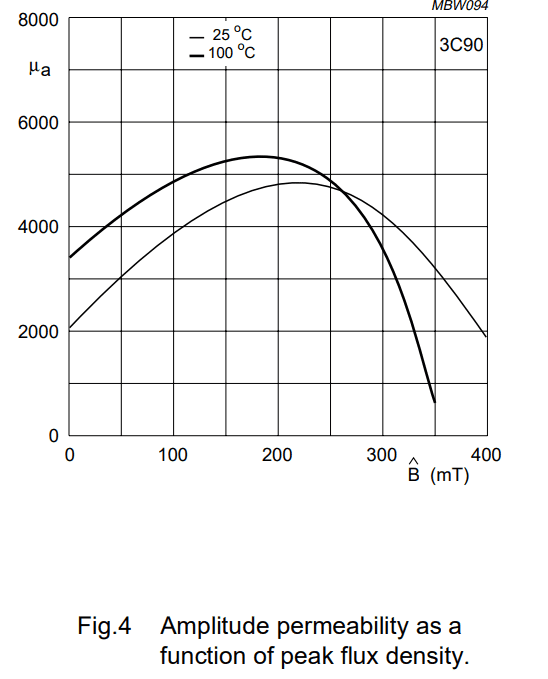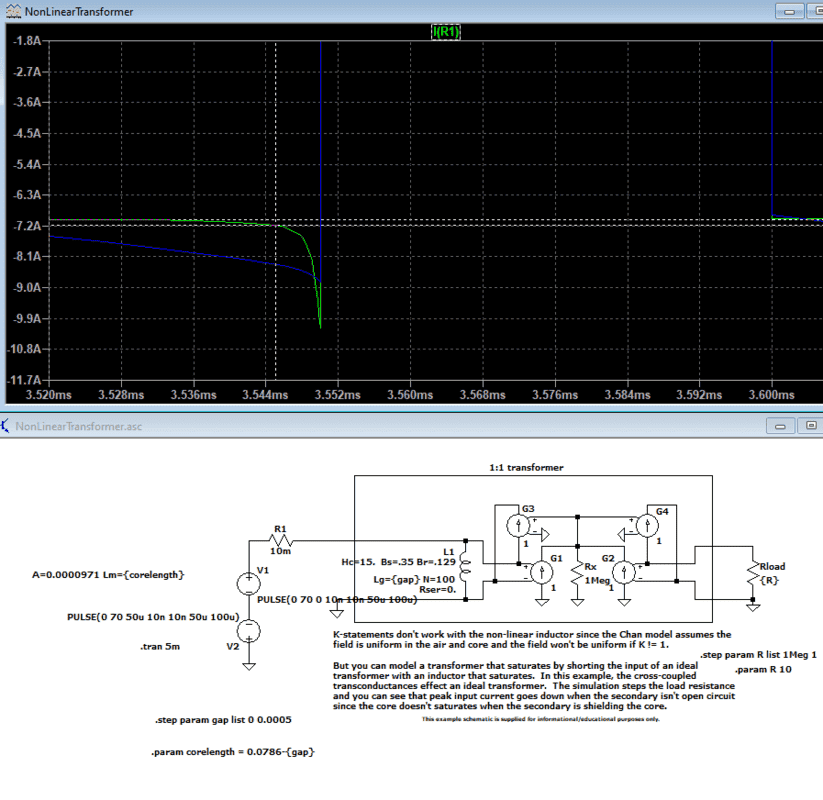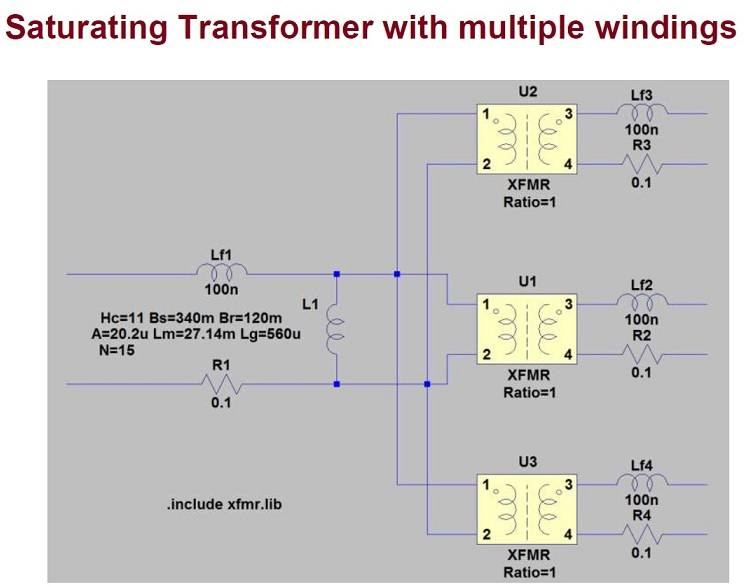# Solving the operating point of a transformer with a nonlinear B-H curve

Trafi
TL;DR Summary
Methods to solve operating point of transformer with nonlinear B-H curve and possibly an air gap
What are the possible ways of solving the operating point of air gapped transformer with nonlinear B-H curve? For example let's consider 3C90 E34 sized core with 0.5 mm airgap. I know that the magnetomotive force over the ferrite part can be formulated as function of the reluctances: ##F_{m,ferrite} = F_{m,tot} * R_{ferrite}/(R_{ferrite}+R_a)##, where ##R_{ferrite}## is the reluctance of the ferrite part and ##R_a## is the reluctance of the air gap. The problem that I do not know how to solve is the fact the ##R_{ferrite}## is also a nonlinear function of the ##F_{m,ferrite}##. I guess this calls for some sort of iterative solution. The only solution method I could think of would be to make piecewise linear approximation from the table of permeability vs Fm and make a guess about the operating point, calculate the ##R_{ferrite}(B)##, recalculate ##F_{m,ferrite}## and do this in a loop until the solution converges.

However, there is another problem. The datasheets does not give the the permeability as a function of field intensity but only as a function of something called peak flux density as show in the attached picture. I could of course turn the reluctance and magnetomotive force into flux but I guess that for my solution I would need the permeability of a small ac excitation on top of a large dc offset. I do not know if that is the case but amplitude permeability as a function of peak flux density sounds to me like it would be the average permeability over large signal sine wave excitation with peak value shown in the X-axis. If this is the case would I need to solve the permeability as a function of field intensity by differentiating the B-H curve?What are the standard ways to solve these type of equations and how could one solve the flux of a real transformer as a function of MMF using the datasheet of the ferrite core? Also making the piecewise linear approximation by hand seems like quite laborious task and I wonder if there are some tools available for these kind of situations for example in octave or as python library. Maybe instead of piecewise linear approximation some sort of curve fitting would be better? Also I want to emphasize that I am especially interested in the nonlinear region near saturation so just approximating the curve with single linear straight curve is not adequate.

ETD34 3C90 datasheet: http://www.farnell.com/datasheets/1468408.pdf
3C90 material datasheet: https://elnamagnetics.com/wp-conten...ube-Materials/3C90_Material_Specification.pdf

Last edited:

alan123hk
Fm,ferrite=Fm,tot∗Rferrite/(Rferrite+Ra), where Rferrite is the reluctance of the ferrite part and Ra is the reluctance of the air gap. The problem that I do not know how to solve is the fact the Rferrite is also a nonlinear function of the Fm,ferrite. I guess this calls for some sort of iterative solution. The only solution method I could think of would be to make piecewise linear approximation from the table of permeability vs Fm and make a guess about the operating point, calculate the Rferrite(B), recalculate Fm,ferrite and do this in a loop until the solution converges.

Yes, you have established a nonlinear magnetic circuit equation. Of course, you can use numerical methods to solve the nonlinear magnetic circuit equation, but as you said, this can be a difficult, tedious and time-consuming task.

However, there is another problem. The datasheets does not give the the permeability as a function of field intensity but only as a function of something called peak flux density as show in the attached picture. I could of course turn the reluctance and magnetomotive force into flux but I guess that for my solution I would need the permeability of a small ac excitation on top of a large dc offset. I do not know if that is the case but amplitude permeability as a function of peak flux density sounds to me like it would be the average permeability over large signal sine wave excitation with peak value shown in the X-axis. If this is the case would I need to solve the permeability as a function of field intensity by differentiating the B-H curve?

I agree with you, in this case, you should use incremental permeability instead.

What are the standard ways to solve these type of equations and how could one solve the flux of a real transformer as a function of MMF using the datasheet of the ferrite core? Also making the piecewise linear approximation by hand seems like quite laborious task and I wonder if there are some tools available for these kind of situations for example in octave or as python library. Maybe instead of piecewise linear approximation some sort of curve fitting would be better? Also I want to emphasize that I am especially interested in the nonlinear region near saturation so just approximating the curve with single linear straight curve is not adequate.

I don't know any standard way to solve these nonlinear magnetic circuit equations, but I will try to use the free software LTspice to simulate and find out the answer. Please note that LTspice provides a nonlinear inductor model, I believe we can further use two or more nonlinear inductors to build a nonlinear transformer.•Trafi
Trafi
Yes there are different levels of nonlinear models available in LTspice. There is the example 32 NonLinearTransformer.asc shipped with LTspice using the CHAN model. I was hoping that solving the actual nonlinear problem even only with numerical methods could give more insight or at least teach new tools to use with other nonlinear problems too. However, you are correct that LTspice will probably be the best way to get results fast with the smallest amount of work. I'll try to plug my values into the CHAN model and report back with results.

Trafi
Here are the results. LTspice has the additional benefit that I can easily study the effect of different design parameters (e.g. airgap size) on the saturation behavior by stepping parameters. In this screencap I am observing the primary current behavior when going into saturation with airgap of 0.0005 m and zero airgap.•alan123hk and dlgoff
alan123hk
Oh, I don't know that LTspice does not support direct coupling between nonlinear inductors using K statements. This is a bit disappointing. Fortunately, we can use other methods to achieve the goal.